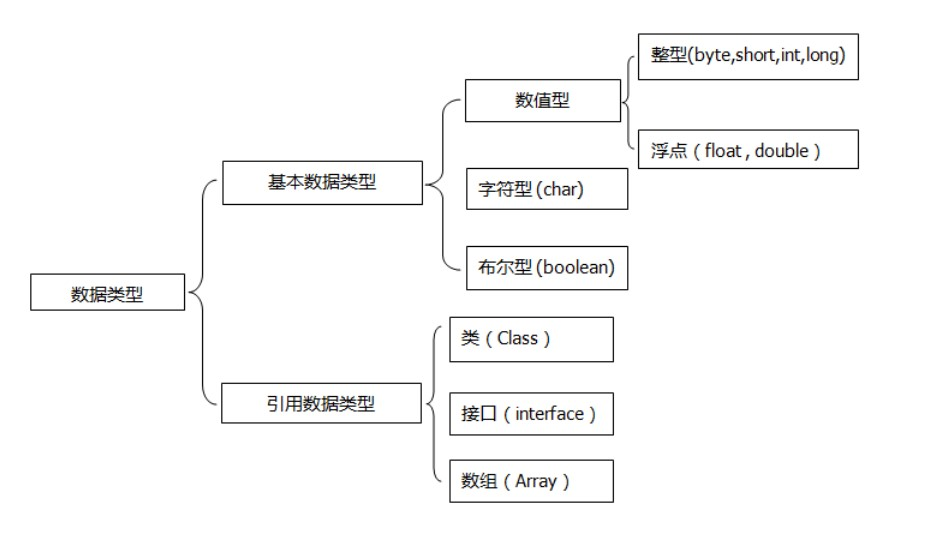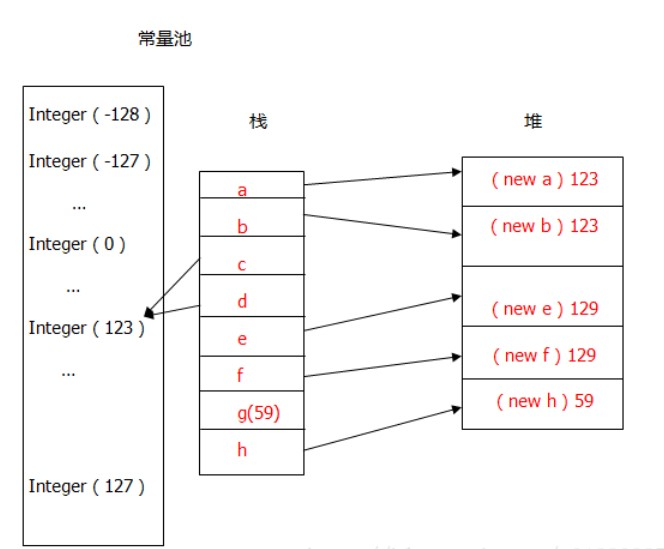# Java深耕之自动装箱拆箱机制

· 8 min read

## 自动装箱与拆箱的定义​

Java中的数据类型分为两类：一类是基本数据类型，另一类是引用数据类型int32Integer
byte8Byte
long64Long
float32Float
double64double
char16Character
boolean1Boolean

## 上自动装箱代码​

``public static void main(String[] args) {        // TODO Auto-generated method stub        int a=3;        //定义一个基本数据类型的变量a赋值3        Integer b=a;        //b是Integer 类定义的对象，直接用int 类型的a赋值            System.out.println(b);        //打印结果为3    }``

``public static Integer valueOf(int i) {               if (i >= -128 && i <= 127)            return IntegerCache.cache[i + 127];            //如果i的值大于-128小于127则返回一个缓冲区中的一个Integer对象        return new Integer(i);        //否则返回 new 一个Integer 对象    }``

## 上自动拆箱代码​

``public static void main(String[] args) {        // TODO Auto-generated method stub        Integer b=new Integer(3);        //b为Integer的对象        int a=b;        //a为一个int的基本数据类型        System.out.println(a);        //打印输出3。    }``

``public int intValue() {        return value;    }``

``public Integer(int value) {        this.value = value;    }``

``public static void main(String[] args) {        // TODO Auto-generated method stub        Integer b=new Integer(3);        //b为Integer的对象        int a=b.intValue();        //其中b.intValue()返回实例化b时构造函数new Integer(3);赋的值3。        System.out.println(a);        //打印输出3。    }``

## 范围概念​

``public static void main(String[] args) {        Integer a = 1000,b=1000;        Integer c=100,d=100;        System.out.println(a==b);        System.out.println(c==d);    }``

``public static void main(String[] args) {                //1        Integer a=new Integer(123);        Integer b=new Integer(123);        System.out.println(a==b);//输出 false        //2         Integer c=123;        Integer d=123;          System.out.println(c==d);//输出 true        //3        Integer e=129;        Integer f=129;        System.out.println(e==f);//输出 false        //4        int g=59;        Integer h=new Integer(59);        System.out.println(g==h);//输出 true    }````public static Integer valueOf(int i) {               if (i >= -128 && i <= 127)            return IntegerCache.cache[i + 127];            //如果i的值大于-128小于127则返回一个缓冲区中的一个Integer对象        return new Integer(i);        //否则返回 new 一个Integer 对象    }``

`` private static class IntegerCache {        static final Integer cache[];        //定义一个Integer类型的数组且数组不可变        static {          //利用静态代码块对数组进行初始化。                                 cache = new Integer;            int j = -128;            for(int k = 0; k < cache.length; k++)                cache[k] = new Integer(j++);        }  //cache[]原来是一个Integer 类型的数组（也可以称为常量池），value 从-128到127，    public static Integer valueOf(int i) {           if (i >=-128 && i <= 127)                    return IntegerCache.cache[i + (-IntegerCache.low)];            //如果装箱时值在-128到127之间，之间返回常量池中的已经初始化后的Integer对象。        return new Integer(i);        //否则返回一个新的对象。    }}``